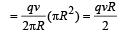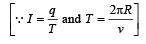Courses

# 28 Year NEET Questions: Moving Charges and Magnetism- 2

## 25 Questions MCQ Test Physics Class 12 | 28 Year NEET Questions: Moving Charges and Magnetism- 2

Description
This mock test of 28 Year NEET Questions: Moving Charges and Magnetism- 2 for NEET helps you for every NEET entrance exam. This contains 25 Multiple Choice Questions for NEET 28 Year NEET Questions: Moving Charges and Magnetism- 2 (mcq) to study with solutions a complete question bank. The solved questions answers in this 28 Year NEET Questions: Moving Charges and Magnetism- 2 quiz give you a good mix of easy questions and tough questions. NEET students definitely take this 28 Year NEET Questions: Moving Charges and Magnetism- 2 exercise for a better result in the exam. You can find other 28 Year NEET Questions: Moving Charges and Magnetism- 2 extra questions, long questions & short questions for NEET on EduRev as well by searching above.
QUESTION: 1

### When a proton is accelerated through 1 V, thenits kinetic energy will be 

Solution:

Potential difference (V) = 1V, K.E. acquired = qV = 1.6 × 10–19 × 1
= 1.6 × 10–19  joules = 1 eV

QUESTION: 2

### If a long hollow copper pipe carries a current,then magnetic field is produced 

Solution:

Inside a hollow pipe carrying current, the magnetic field is zero, since according to Ampere's law, Bi. 2πr = μ0 × 0 ⇒ Bi = 0.
But for external points, the current behaves as if it was concentrated at the axis only; so, outside,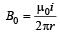Thus, the magnetic field is produced outside the pipe only.

QUESTION: 3

### Two long parallel wires P and Q are both perpendicular to the plane of the paper with distance of 5 m between them. If P and Q carry currents of 2.5 amp and 5 amp respectively in the same direction, then the magnetic field at a point half-way between the wires is       

Solution:

When the current flows in both wires in the same direction then magnetic field at half way due to the wire P,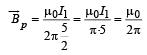(where I1= 2.5 amp) The direction of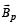is downward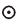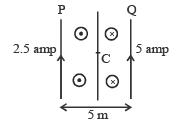Magnetic field at half way due to wire Q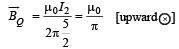[where I2 = 2.5amp.]

Net magnetic field at half way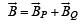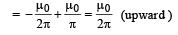Hence, net magnetic field at midpoint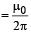QUESTION: 4

A proton moving with a velocity 3 × 105 m/senters a magnetic field of 0.3 tesla at an angle of30º with the field. The radius of curvature of itspath will be (e/m for proton = 108 C/kg)         

Solution: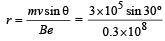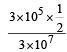= 0.5 x 10 -2m = 0.5cm

QUESTION: 5

In a certain region of space electric field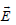and magnetic field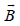are perpendicular to each other and an electron enters in region perpendicular to the direction ofandboth and moves undeflected, then velocity of electron is 

Solution:

Electron moves undeflected if force exerted due to electric field is equal to force due to magnetic field.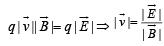QUESTION: 6

A charged particle of charge q and mass m enters perpendicularly in a magnetic field. Kinetic energy of the particle is E; then frequency of rotation is 

Solution:

For circular path in magnetic field, mrω2 = qvB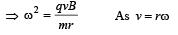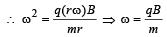∴  If v is frequency of roatation, then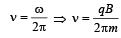QUESTION: 7

A galvanometer can be converted into avoltmeter by connecting           

Solution:

A galvanometer can be converted in to a voltmeter by connecting  the high ressistance in series with the galvanometer so that only a small amount of current passes through it.

QUESTION: 8

A wire carries a current. Maintaining the samecurrent it is bent first to form a circular plane coil ofone turn which produces a magnetic field B at thecentre of the coil. The same length is now bentmore sharply to give a double loop of smaller radius.The magnetic field at the centre of the double loop,caused by the same current is          

Solution:

Let I be current and l be the length of the wire.
For Ist case :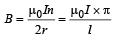where
2πr = l and n = 1

For IInd Case :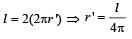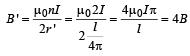QUESTION: 9

A particle having charge q moves with a velocity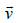through a region in which both an electric fieldand a magnetic fieldare present .The force on the particle is           

Solution:

Force due to electric field =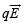Force due to magnetic field =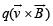Net force experienced =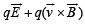QUESTION: 10

A charged particle moves through a magneticfield in a direction perpendicular to it. Then the         

Solution:

Magnetic for ce acts perpendicular to the velocity. Hence speed remains constant.

QUESTION: 11

A long solenoid carrying a current produces amagnetic field B along its axis. If the current isdouble and the number of turns per cm is halved,the new value of the magnetic field is     

Solution:

B = μ0ni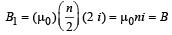⇒ B1 = B

QUESTION: 12

A galvanometer acting as a voltmeter will have            

Solution:

A high resistance is connected in series so that less current passes through voltmeter.

QUESTION: 13

A galvanometer of 50 ohm resistance has 25 divisions. A current of 4 × 10–4 ampere gives adeflection of one per division. To convert thisgalvanometer into a voltmeter having a rangeof 25 volts, it should be connected with aresistance of 

Solution:

Rg = 50Ω, Ig = 25 × 4 × 10–AΩ = 10–2 A
Range of V = 25 volts V = Ig(Re + Rg)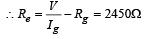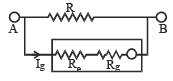QUESTION: 14

An electron moves in a circular orbit with a uniform speed v. It produces a magnetic field B at the centre of the circle. The radius of the circle is proportional to      

Solution:

The magnetic field produce by moving electron in circular path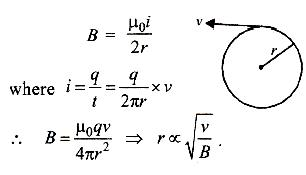QUESTION: 15

A very long straight wire carries a current I. At the instant when a charge + Q at point P has velocity, as shown, the force on the charge is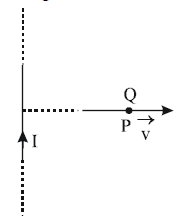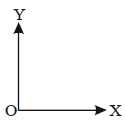Solution:

The direction of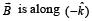∴  The magnetic  force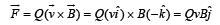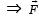is along OY.

QUESTION: 16

When a charged particle moving with velocityis subjected to a magnetic field of inductionthe force on it is non-zero. This implies that

Solution:

Force on a particle moving with velocity v in a magnetic field  B is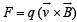If angle between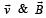is either zero or 180º, then value of F will be zero as cross product of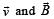will be zero.
So option (b) is correct.

QUESTION: 17

Two circular coils 1 and 2 are made from the samewire but the radius of the 1st coil is twice that ofthe 2nd coil. What potential difference in voltsshould be applied across them so that themagnetic field at their centres is the same 

Solution:

If R1 & R2 be the radius of the circular wires,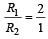If same potential is applied on them, current in Ist will be half that in the later. If V potential is applied on them, current in them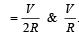Now magnetic field at the centre of circular

coil,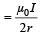For first wire, field B1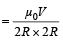For second wire, field B2  =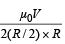Given B1 = B2 The given data do not provide any required result. There is a mistake in the framing of the question.

QUESTION: 18

A beam of electron passes undeflected throughmutually perpendicular electric and magnetic fields.If the electric field is switched off, and the samemagnetic field is maintained, the electrons move  

Solution:

If the electric field is switched off, and the same magnetic field is maintained, the electrons move in a circular orbit and electron will travel a magnetic field ⊥ to its velocity..

QUESTION: 19

In a mass spectrometer used for measuring the masses of ions, the ions are initially accelerated by an electric potential V and then made to describe semicircular path of radius R using a magnetic field B. If V and B are kept constant, the ratio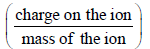will be proportional to

Solution:

In mass spectro meter, when ions are accelerated through potential V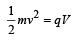..........(i)

As the magnetic field curves the path of the ions in a semicircular orbit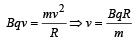.......... (ii)

Substituting (ii) in (i)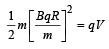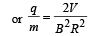Since V and B are constants,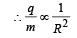QUESTION: 20

Under the influence of a uniform magnetic fielda charged particle is moving in a circle of radiusR with constant speed v. The time period of themotion        

Solution: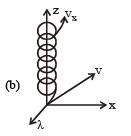When a test charge q0 enters a magnetic field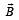directed along z-axis, with a velocity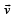making angles d with the z-axis. The time period of the motion is independent of R and v.

QUESTION: 21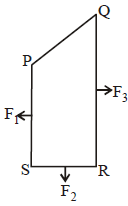A closed loop PQRS carrying a current is placed in a uniform magnetic field. If the magnetic forces on segments PS, SR, and RQ are F1 , F2 and F3 respectively and are in the plane of the paper and along the directions shown, the force on the segment QP is       

Solution:

According to the figure the magnitude of force on the segment QM is F3 –F1 and PM is F2.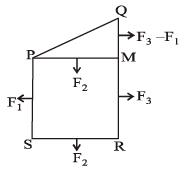Therefore, the magnitude of the force on segment PQ is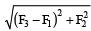QUESTION: 22

A particle of mass m, charge Q and kinetic energyT enters a transverse uniform magnetic field ofinduction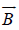. After 3 seconds, the kinetic energyof the particle will be: 

Solution:

When a charged particle enters a transverse magnetic field it traverse a circular path. Its kinetic energy remains constant.

QUESTION: 23

A circular disc of radius 0.2 meter is placed in a uniform magnetic field of induction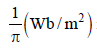in such a way that its axis makes an angle of 60° with. The magnetic flux linked with the disc is:        

Solution:

Here,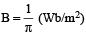θ = 60°
Area normal to the plane of the disc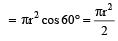Flux = B × normal area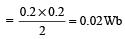QUESTION: 24

A galvanometer having a coil resistance of 60 Ωshows full scale deflection when a current of 1.0amp passes through it. It can be convertedinto an ammeter to read currents upto 5.0 amp by         

Solution:

G = 60Ω, Ig=1.0A, I=5A.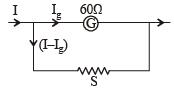Let S be the shunt resistance connected in parallel to galvanometer IgG = (I – Ig) S,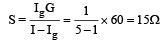Thus by putting 15Ω in parallel, the galvanometer can be converted into an ammeter.

QUESTION: 25

A charged paritcle (charge q) is moving in a circleof radius R with uniform speed v. The associatedmagnetic moment μ is given by 

Solution:

Magnetic moment, m = IA